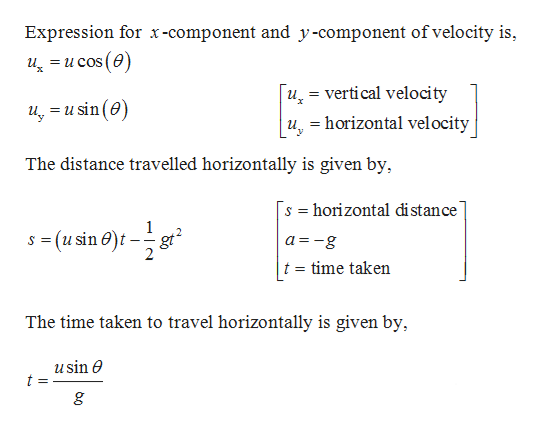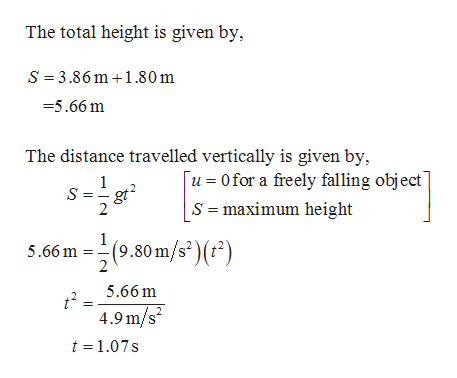# Given the density of iron as 7800 kg/m 3 , and the Earth radius is 6.38 x 10 6 m, if 55% of the Earth's volume is filled with Iron, and 45% is filled with rocks that has a density of 2600 kg/m 3 , what is the total mass of the Earth?

Question
17 views

Given the density of iron as 7800 kg/m 3 , and the Earth radius is 6.38 x 10 6 m, if 55% of the Earth's volume is filled with Iron, and 45% is filled with rocks that has a density of 2600 kg/m 3 , what is the total mass of the Earth?

check_circle

Step 1

Kinematics equation of motion for one dimensional motion with constant acceleration is used to find the distance travelled by the object horizontally. Using this the distance travelled by the shot vertically can be found.help_outlineImage TranscriptioncloseExpression for x-component and y-component of velocity is os (e) ucos u, verti cal velocity u, horizontal velocity = u usin (e) The distance travelled horizontally is given by, s horizontal distance 1 -(usin e)gt a -g t time taken The time taken to travel horizontally is given by, usin e t = g fullscreen
Step 2

Substitute 42 degree for theta, 13.0m/s for u, and 9.8m/s^2 for g in the above equation to find the distance travelled horizontally.

Step 3

The total height will be the sum of the distance travelled hor...help_outlineImage TranscriptioncloseThe total height is given by S 3.86m 1.80m =5.66 m The distance travelled vertically is given by, Tu0for a freely falling object 1 S gt 2 S maximum height 1 5.66 m 2 (9.80 m/s' () 25.66m 4.9 m/s t 1.07s fullscreen

### Want to see the full answer?

See Solution

#### Want to see this answer and more?

Solutions are written by subject experts who are available 24/7. Questions are typically answered within 1 hour.*

See Solution
*Response times may vary by subject and question.
Tagged in

### Physics